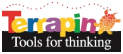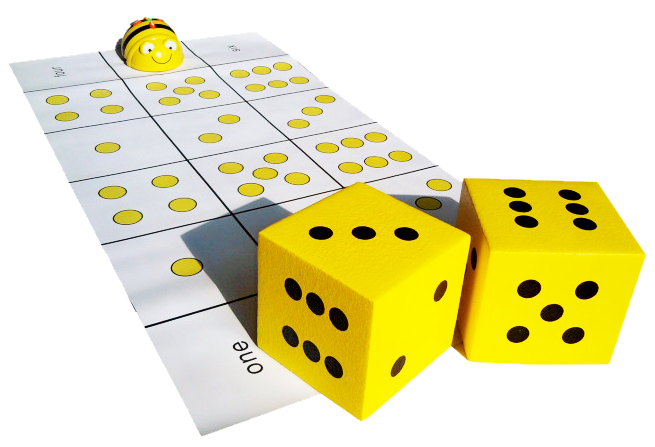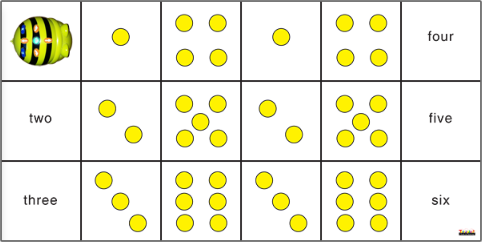Terrapin Resources

### Dice MatView a larger version of the mat.This is the emulator mat.

The Dice Mat and accompanying pair of oversized foam dice inspire many fun games that build counting, matching, and number skills. This mat encourages students to think of different ways to represent numbers.

In addition to dice, which represent amounts using the pips (dots) on the dice, can students think of other ways to represent and compare numbers? If you have them in your classroom, bring out and discuss dominoes (which represent numbers the way dice do), Cuisenaire rods, counting chips, playing cards, hash marks, and so on.

##### Lesson Ideas

Although these ideas refer to Bee-Bot, they can all be done with Blue-Bot as well.

Remind students to press X to erase the commands the previous student entered.

Lesson Idea 1: Matching the Die to its Dots

• Divide students into groups.
• Have each student toss one die and send Bee-Bot to the image that matches the number of pips.
• The next student presses X to clear those commands.
• Repeat the process with the new student.

Lesson Idea 2: Matching the Die to its Number

• Divide students into groups.
• Have each student toss one die and send Bee-Bot to the number word that matches the number of pips.
• The next student presses X to clear those commands.
• Repeat the process with the new student.

Lesson Idea 3: Rolling Both Dice

• Divide students into groups.
• Have each student roll both dice.
• The student then navigates Bee-Bot to the two pictures for what is shown on the dice, with a Pause in between.

Lesson Idea 4: Adding Up the Numbers

• Divide students into groups.
• Have a student roll the dice.
• Have students figure out how many different paths Bee-Bot can take to go to numbers that add up to that total? Add a Pause between stops.

For example, if the dice total ten, the paths could be:

• 5 + 5
• 5 + 3 + 2
• 4 + 6
• 2 + 3 + 2 + 3
• etc.

Lesson Idea 5: Using the Online Dice Mat

If students are using computers in your classroom, have them use the online Dice Mat in the Bee-Bot Emulator in a browser. They can explore the mat using these Lesson ideas.

Lesson Idea 6: Inventing a Game

Encourage students to make up their own game using this mat and the foam dice.

##### Mat Details

This mat is incorporated into several of the Bee-Bot Lessons. These lessons give teachers lesson plans that are tied to national curriculum standards. They give students an opportunity to engage in over 180 learning activities using the floor Bee-Bot or Blue-Bot or the same activities in the online emulator.

The mat is made of washable vinyl and measures approximately 18” by 36” (45 cm by 90 cm)

View the mat in Terrapin’s store.